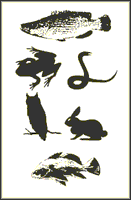## Nebraska Cooperative Fish & Wildlife Research Unit## Nebraska Cooperative Fish & Wildlife Research Unit -- Staff Publications

1995

#### Citation

Journal of Freshwater Ecology, Volume 10, Number 2

#### Abstract

Weight-length data were compiled trom 289 redear sunfish Lepomis

microlophus populations. We applied the regression· line-percentile (RLP)

technique to weight-length data for 150 redear sunfish populations to develop

a new 75th-percentile standard weight (Ws) equation. The proposed RLP Ws

equation, log10Ws = -4.968 + 3.119log10TL where Ws is the standard weight

In grams and TL is the total length in millimeters, is valid for fish ≥70 mm TL

The English-unit equivalent, log10Ws = -3.263 + 3.119log10TL where Ws is the

standard weight In pounds and TL is the total length in inches, Is valid for fish

~3 in TL. We used the remaining 139 independent populations to evaluate the

current [log10 Ws = -5.164 + 3.227(log10TL)] and RLP Ws equations for any

length-related biases. We confirmed that the current Ws equation is length-biased.

That is, relative weight (Wr) values significantly decreased with

increasing fish length for far more populations (N=45) than they increased

(N=7). We tested the proposed RLP Ws equation with the same 139

populations, and found no consistent length-related bias in Wr values

calculated with the proposed RLP Ws equation (N=38, 29). Thus, we

recommend the use of the proposed RLP Ws equation for redear sunfish.

COinS